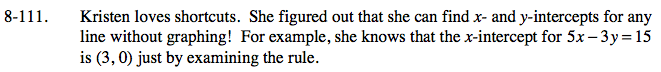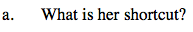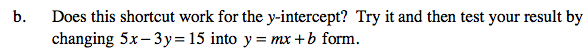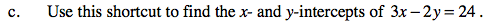### Home > CAAC > Chapter 8 > Lesson 8.3.2 > Problem8-111

8-111.
1. Kristen loves shortcuts. She figured out that she can find x- and y-intercepts for any line without graphing! For example, she knows that the x-intercept for 5x − 3y = 15 is (3, 0) just by examining the rule. Homework Help ✎

1. What is her shortcut?

2. Does this shortcut work for the y-intercept? Try it and then test your result by changing 5x − 3y = 15 into y = mx + b form.

3. Use this shortcut to find the x- and y-intercepts of 3x − 2y = 24.She knew the x-intercept occured when y = 0.5(0) − 3y = 15

$y = -\frac{5}{3}x - 5$3x − 2(0) = 24 3(0) − 2y = 24